## Written methods of multiplication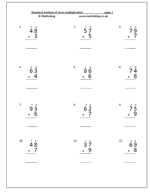In our Four Rules, Multiplication category we have a range of pages which look at written methods of multiplication. Parents seem to have been confused by what is taught in school, but it is really the process leading up to the standard written method which has changed: the standard method itself is very much the same as when parents were children and even when grandparents were children!

The worksheets take a brief look at moving towards the standard method and then we have worksheets on:

• short multiplication: multiplying 2-digits by 1-digit
• multiplying 2-digit numbers by multiples of 10
• multiplying 3-digits by 1-digit
• multiplying money by a single digit eg £3.45 x 4
• multiplying decimals eg 3.8 x 7

On most of these pages there are clear explanations of how to do them, which have been very popular with both parents and teachers.

Why not go to our Four Rules, Written Multiplication section?

## What maths should children know by the end of Year 5?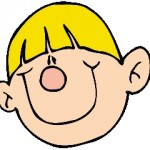Much of the work carried out in year 4 eg addition and subtraction is reinforced in year 5. Efficient written methods for all addition, subtraction and multiplication are expected and moving towards this with division.
Please note that this is only a summary of the key areas of maths to be covered in the year.

Counting and understanding number:

By the end of year 5 children should
• read and write larger numbers.

• count back beyond zero.
(eg count back 7 from 3.)

• count on and back in decimal steps.
(eg count on 6 tenths from 4.7)

• explain what each digit represents in whole numbers.
(eg say what the value of the 7 is in 347 450.)

• explain what each digit represents in decimals up to two places.
(eg say what the value of the 7 is in 34.75)

• partition numbers.
(eg 4.56 = 4 units + 5 tenths + 6 hundredths.)

• round whole numbers.
(eg round 3 501 to the nearest thousand.)

• order decimals.
(eg which is bigger 4.3 or 4.09?)

• write a smaller whole number as a fraction of a larger one.
(eg recognise that 7 out of 10 is 7/10.)

• find equivalent fractions.
(eg 6/10 = 12/20.)

• relate fractions to decimals.
(eg know that 7/10 = 0.7)

• begin to understand percentage.
(eg know that 40% means 40 out of 100 or 40/100.)

• begin to solve problems using ratio and proportion.
(eg a chicken must be cooked for 50 minutes for every kilo. How long must a 3 kilo chicken be cooked?)

Knowing and using number facts:

By the end of year 5 children should
• use their knowledge to add two decimals mentally.
(eg 7.5 + 3.6)

• use their knowledge to subtract two decimals mentally.
(eg 7.5 – 3.4)

• use their knowledge to double or halve two decimals mentally.
(eg double 5.7)

• recall rapidly all tables up to 10 x 10.
(eg 9 x 7.)

• use knowledge of tables to multiply pairs of multiples of 10 or 100.
(eg 90 x 70.)

• use knowledge of tables to derive division facts.
(eg 800 divided by 4.)

• find pairs of factors of a number.
(eg find the factors of 15.)

• find common multiples of two numbers.
(eg find the common multiples of 9 and 12.)

• use knowledge of number to estimate and check answers.
(eg know that 36 x 19 is about 35 x 20.)

Calculating:

By the end of year 5 children should
• respond rapidly to oral and written questions using addition and subtraction.
(eg increase 190 by 24.)

• use efficient (standard) methods for addition, including decimals.

• use efficient (standard) methods for subtraction, including decimals.
(eg subtract 368 from 4134.)

• extend mental calculations to multiply a 2-digit number by a single digit
(eg 16 x 5.)

• extend mental calculations to multiply a 2-digit number by a 2-digit number
(eg 16 x 25.)

• use understanding of place value to multiply numbers by 10, 100 or 1000.
(eg multiply 4.5 by 100.)

• refine written methods of multiplication to multiply HTU by U, TU by TU, U.t by U.
(eg multiply 24 by 35.)

• refine written methods of division to divide HTU by U.
(eg divide 374 by 5.)

• find fractions using division.
(eg find 1/100 of an amount by dividing by 100.)

• Find percentages of numbers and quantities.
(eg find 15% of £40.)

• Use a calculator to solve problems involving larger numbers, decimals and fractions.
(eg find ¾ of 450g.)

Understanding shape:

By the end of year 5 children should
• be able to recognise and describe the properties of rectangles, triangles, regular polygons and 3-D solids.
(eg recognise that rectangles have four right angles.)

• identify and draw nets of 3-D solids.
(eg find different nets for an open cube.)

• read and plot co-ordinate points.
(eg know the convention (3,2) for describing a point.)

• recognise parallel and perpendicular lines in shapes and use a set square and ruler to draw shapes.

• draw the position of a simple shape after reflection or translation.

• estimate, draw and measure acute angles and obtuse angles using a protractor.
(eg draw accurately an angle of 60 degrees.)

• calculate angles in a straight line.
(eg use knowledge that an angle of 180 degrees is a straight line.)

Measuring:

By the end of year 5 children should
• use standard metric units of length, weight and capacity.

• convert larger units to smaller units using decimals to one place.
(eg change 3.7 kg to 3 700g.)

• correctly take a reading that lies between two numbered divisions on a scale.
• draw and measure lines to the nearest millimetre.
(eg draw a line 3.6 cm long to within one millimetre accuracy.)

• measure and calculate the perimeter of regular and irregular polygons.
(eg measure one side of a regular pentagon and multiply by 5 to calculate the perimeter.)

• use the formula for the area of a rectangle.
(work out the area of a rectangle 6 cm long and 4 cm wide)

• read timetables and use the 24 hour clock.
(convert 7:00 pm to 19:00 h.)

Handling data:

By the end of year 5 children should
• use the language of probability to describe events, including: fair, unfair, likely, unlikely, certain, uncertain, probable, possible, impossible.
(eg “It is likely that I will watch TV tonight.”)

• answer questions by collecting, sorting and organising data and identify further questions to ask.
(eg test the statement: “You are just as likely to throw a 6 as any other number on a dice.”)

• construct frequency tables, pictograms, bar and line graphs.
(eg draw a line graph showing room temperature over time.)

• find and interpret the mode of a set of data.
(eg find the most common item in a list.)

Using and applying mathematics:

By the end of year 5 children should
• solve one-step and two-step problems involving money, measures, time, and numbers by choosing appropriate calculations, including decimals and calculators.
(eg decide what calculations to carry out to reach an answer.)

• solve puzzles and present the solution.
(eg arrange the numbers in a magic square.)

• suggest a line of enquiry and collect the necessary information to find the answer.
(eg describe a short way to work out the area of a rectangle.)

• make a statement and test it with examples.
(eg angles on a straight line add up to 180 degrees.)

## Adding fractions with totals more than one.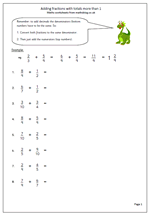One of the hardest things to get across to children is how to add fractions. All too often they will just add the numerator (top number of the fraction) and denominator (bottom number of the fraction) and get the wrong answer. The key to success is to create two equivalent fractions with the same denominator. The denominator stays the same when adding the numerators.

Look at 2/3 + 5/9

It is not possible to add thirds and ninths directly, so the two fractions need to be converted to their lowest common denominator; in this case ninths. Don’t try to add fractions until children have a really good understanding of equivalent fractions.

2/3 is the same as 6/9 so the question now becomes:

6/9 + 5/9 which is easy: 6/9 + 5/9 = 11/9

Convert the 11/9 to a mixed number which is 1 and 2/9.

Done!

Adding fractions that total more than 1

## Year 5: converting metric units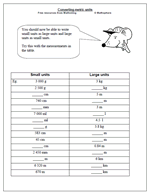Just a quick exercise in converting metric units from small to large and vice versa. This is not easy and as stated before a good understanding of both the metric system and place value is needed to be able to do this.
It is probably easier to convert large units to smaller:
Eg 3.5 litres = 3 500 ml
Converting small to large can bring problems with place value
Eg 740 cm = 7.4 metres or 7.40 metres. Either is correct.
By now children should know all the facts about the metric system and there is not a lot of point in attempting this if they don’t.

## 7 times table practice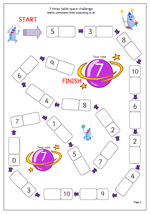I have had several requests for more in the way of tables practice sheets: so here is one for the 7 times table.

The 7 times table is probably the hardest of the lot as there is little in the way of a recognisable pattern in the digits. However, if 2, 3, 4, 5 6 and 10 times tables have already been learned, then there is not much else to worry about!

Use this sheet as a timed exercise or as a check to see if the table is really well know.

It can be found in our Four Rules category, under mental methods of multiplication.

7x tables space challenge

## What maths should children know by the end of Year 4?Now we have come to what is expected of children aged between 8 and 9: as defined by the English Primary Framework for Mathematics (part of the National Curriculum). In year 4 there is a greater emphasis on written methods, with the standard or efficient method expected for both addition and subtraction. Children are expected to know all their tables and there is a lot more work on decimals.

Counting and understanding number:

By the end of year 4 children should
• read and write whole numbers up to thousands.
(eg write in words 4009.)

• be able to continue number sequences counting on and back in even steps.
(eg count back 60 in tens from 645.)

• partition 4-digit numbers into thousands, hundreds, tens and units.
(eg know that 2547 is 2 000 + 500 + 40 + 7.)

• order and round 4-digit numbers.
(eg round 3456 to the nearest hundred.)

• use negative numbers and place them on a number line.
(eg reading a thermometer below zero.)

• state inequalities using < and > signs.
(eg -4<0)

## Maths worksheets on Knowing Multiplication Facts in year 6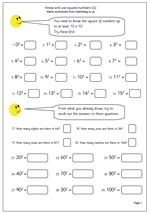Expectations of what children should know are high in Year 6 but we have a great selection of maths worksheets to help. It is assumed (often wrongly) that by Year 6 children have a good knowledge of ‘tables’ and can quickly recall any table from 2 to 10. With this in mind they can put their knowledge to good use by multiplying decimals mentally. A question such as 0.6 x 5 is not that much trickier than 6 x 5, as long as place value is understood.

Square numbers are a part of this ‘tables’ knowledge, leading to questions such as what is the square of 30? It is worth spending a little time making sure that children know their square numbers up to 12 x 12 as they will occur time and time again when moving onto high school maths.

## 6 SATs practice questions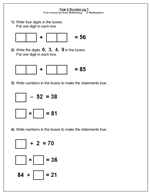This is the first in my series of ‘Booster’ worksheets for year 6 children who are about to take the SAT tests. They aim to boost a child’s score in the test papers by making them familiar with the types of questions that they will come across.

It is rare for a straightforward sum of the type 34 + 67 = to appear on the paper. Usually sums are in the form of number sentences with numbers missing. Often there can be more than one correct answer.

For example, if you look at the first question, there are many possible answers. A tip here is to keep it simple. Why not write 46 + 10? Perfectly correct!

Plenty more like this in the Year 6 worksheets category

Booster_pg 1

## Year 4 quite hard mental subtraction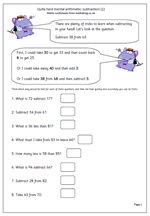Many children are unaware that there are often several ways of working out a calculation and that those who choose the best methods find maths easier and are able to answer questions more quickly and more accurately.

Let’s look at ‘Subtract 38 from 63.

This should be done, ‘in your head’ and will involve several stages, depending on the method used.

First, I could take 30 from 63 to leave 33. Then I could count back 8 from 33 which gives me 25.

Secondly, I could take 40 from 63 leaving 23 and then compensate by adding 2, which gives me 25.

Thirdly, I could take 38 from 68, leaving 30 and then compensate by subtracting 5 (because 63 is 5 less than 68).

There are several other ways very similar to these, but this does show that there is no ‘one right way, when it comes to mental arithmetic.

Quite hard mental arithmetic:_subtraction (1)

## Written subtraction of 3-digits (2)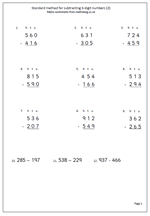Written subtraction is one of those areas of maths that children frequently get wrong, mainly because they do not fully understand the method. This page looks at 3-digit subtraction and all the questions need  ‘decomposition’ in order to get them correct. The last three questions should be laid out in the same way as the questions above.

Watch out for when there is a zero on the top line; for example when faced with question one:

zero subtract six  is not six.

Rather, say ‘zero take way six cannot be done so I need to borrow 10 from the tens column.’ (I don’t really like this phrase because zero take away six can be done: the answer is negative six, but this takes us into a completely different method of subtraction which I will discuss one day when  it’s been raining all day!)

It is always a good idea to check the answer by adding the answer line to the line above, which, in turn should give you the number at the top of the question.

This page can be found in our Four Rules section, under subtraction.

Standard subtraction of_3_digits_(2)